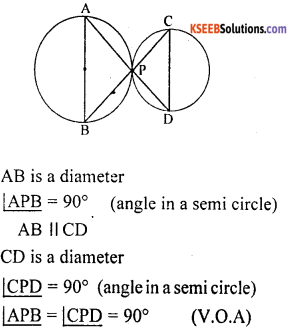# KSEEB Solutions for Class 10 Maths Chapter 4 Circles Additional Questions

Students can Download Class 10 Maths Chapter 4 Circles Additional Questions Questions, Notes Pdf, KSEEB Solutions for Class 10 Maths helps you to revise the complete Karnataka State Board Syllabus and score more marks in your in examinations

## Karnataka State Syllabus Class 10 Maths Chapter 4 Circles Additional Questions

I. Multiple Choice Questions:

Question 1.
Line segment joining the centre and a point on the circle is called
(b) diameter
(c) Chord
(d) Arc

Question 2.
Part of a circle is called
(a) Chord
(b) diameter
(c) Segment
(d) Arc
(d) ArcQuestion 3.
The biggest chord in a circle is called
(b) diameter
(c) chord
(d) Arc
(b) diameter

Question 4.
The region, bounded by a major arc and a chord is called
(a) Segment
(b) major segment
(c) minor segment
(d) major arc
(b) major segment

Question 5.
The length of the biggest chord is 8 cm then the value of radius is
(a) 8 cm
(b) 4 cm
(c) 3 cm
(d) 5 cm
(b) 4 cm

Question 6.
How many radius can be drawn in circle
(a) 1
(b) 2
(c) only 3
(d) many
(d) many

Question 7.
An angle in a semicircle is.
(a) 60°
(b) 30°
(c) 90°
(d) 180°
(c) 90°

Question 8.
Equal chords of a circle are.
(a) Equidistant from the centre.
(b) Equal
(c) Unequal
(d) Not equidistant from the centre
(b) Equal

Question 9.
If the length of the chord increases its perpendicular distance from the centre.
(a) Increases
(b) Decreases
(c) Equal
(d) Constant
(b) Decreases

Question 10.
The perpendicular distance between the biggest chord and the centre is.
(a) zero
(b) Equal
(c) 9 cm
(d) 10cm
(a) zero

Question 11.
In a circle angles in the major segment are called.
(a) Obtuse angles
(b) Acute angles.
(c) Right angles
(d) Complete angle
(b) Acute angles.Question 12.
In a circle angles in the minor segment are called.
(a) Obtuse angles
(b) Acute angles.
(c) Right angles
(d) zero angle
(a) Obtuse angles

Question 13.
In a circle angles in the same segment are
(a) Not equal
(b) Right angles
(c) Equal
(d) zero angle.
(c) Equal

Question 14.
Circles having the same centre but different radii are called.
(a) Congruent circles
(b) Concentric circles
(c) Equal circles
(d) None of these
(b) Concentric circles

Question 15.
Circles having same radii but different centres are called
(a) Congruent circles
(b) Concentric circles
(c) Equal circles.
(d) Intersecting circles
(a) Congruent circles

Question 16.
The number of circles are drawn through three non-collinear points in a plane is.
(a) 1
(b) 2
(c) 3
(d) 4
(a) 1

Question 17.
A line which intersects a circle in two points is called
(a) A secant
(b) A chord
(c) An arc
(d) A tangent
(a) A secant

Question 18.
A line which intersects a circle in only one point is called
(a) A secant
(b) A tangent
(c) A chord
(d) A diameter
(b) A tangent

Question 19.
A tangent to a circle intersects the circle is
(a) one point only
(b) Two points
(c) No point
(d) Three points
(a) one point only

Question 20.
A secant of a circle intersects the circle in
(a) only one point
(b) Two points
(c) Three points
(d) No point
(b) Two points

Question 21.
The point where a tangent line intersects a circle is called the
(a) centre
(b) point of contact
(c) End-point
(d) None of these
(b) point of contactQuestion 22.
The angle between the tangent at any point of a circle and the radius through the point of contact is
(a) 60°
(b) 90°
(c) 45°
(d) 30°
(b) 90°

Question 23.
How many tangents can be drawn to a circle at any point of it?
(a) 1
(b) 2
(c) 3
(d) None of these
(a) 1

Question 24.
How many parallel tangents can a circle have at the most?
(a) 1
(b) 2
(c) 4
(d) 3
(b) 2

Question 25.
Two circles of radii 5 cm and 3 cm touch each other externally. The distance between their centres is
(a) 5 cm
(b) 3 cm
(c) 2 cm
(d) 8 cm
(d) 8 cm

Question 26.
Two circles of radii 5 cm and 3 cm touch each other internally. The distance between their centres is
(a) 5 cm
(b) 3 cm
(c) 2 cm
(d) 8 cm
(c) 2 cm

Question 27.
The tangents at the endpoints of a diameter of circle are
(a) perpendicular
(b) parallel
(c) intersecting
(d) inclined at 60°
(b) parallel

Question 28.
The length of the tangent from a point A at distance 5 cm from the centre of the circle is 4 cm. The radius of the circle is
(a) 3 cm
(b) 2 cm
(c) 5 cm
(d) 4 cm
(a) 3 cm

Question 29.
Two concentric circles of radii 5 cm, and 3 cm. Find the length of the chord of the larger circle which touches the smaller circle.
(a) 8 cm
(b) 6 cm
(c) 4 cm
(d) 10 cm
(a) 8 cm

Question 30.
How many tangent lines can be drawn to a circle from a point outside the circle?
(a) 1
(b) 2
(c) 3
(d) 4
(b) 2Question 31.
How many tangents can be drawn to a circle have
(a) 2
(b) infinitely many
(c) one
(d) no
(b) infinitely many

Question 32.
In the following figure, |PBA is
(a) 60°
(b) 30°
(d) 45°
(d) none of these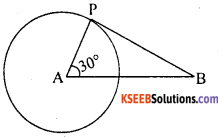(a) 60°

Question 33.
In the following figure, Find AP if AB = 5 cm
(a) 5 cm
(b) 4 cm
(c) 3 ccm
(d) 2 cm(a) 5 cm

Question 34.
In the following figure, find the perimeter of A APQ if AB = 6 cm.
(a) 10 cm
(b)12cm
(c) 15 cm
(d) 6 cm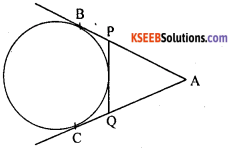(b)12cm

Question 35.
In the following Figure, find the length of the chord AB if PA = 4 cm and OP = 3 cm.
(a) 2 cm
(b) 4 cm
(c) 10cm
(d) 5 cm(c) 10cm

Question 1.
Define concentric circles.
Circles which are having same centre and different radii are called concentric circles.

Question 2.
Define congruent circles.
Circles which are having same radii & different centres are called congruent circles.

Question 3.
Define the sector of a circle.
The region bounded by an arc of a circle and its two bounding radii is called sector.

Question 4.
Name the biggest chord of a circle.
Diameter

Question 5.
Write the formula to find the perimeter of a circle.
Circumference = C = 2nx.

Question 6.
Name the angle formed in a semi-circle is
Right angleQuestion 7.
Name the angle formed in a major segment.
Acute angle

Question 8.
Name the angle formed in a minor segment.
Obtuse angle.

Question 9.
The length of the tangent to a circle from a point P, which is 25 cm away from the centre, is 24 cm. What is the radius of the circle?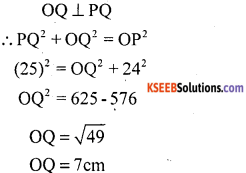Question 10.
In a quadrilateral, ABCD circumscribes a circle with centre O. If ∠AOB = 110°, then find ∠COD.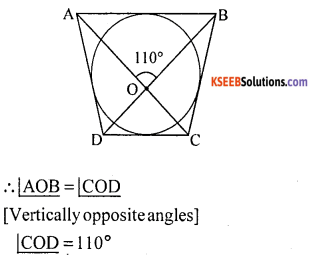Question 11.
Write the relationship between radius and diameter.
d = 2r (or) r = d/2

Question 12.
Angles formed in a same segment are ________
Equal

Question 1.
In the following figure if ∠DAB = 60°
and ∠ACB = 70°, find the measure of ∠DBA.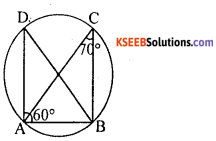Question 2.
Prove that the perpendicular from the centre of a circle to a chord, bisect the chord.AB is the chord of a circle with centre O and OD ⊥ AB. We have to prove that AD = DB
In ∆ ODA and ∆ ODB.
OD = OD (common)
∠ODA = ∠ODB = 90° (OD ⊥ AB)
∆ ODA = ∆ ODB (R H S)Question 3.
If two equal chords of a circle intersect within the circle, prove that the segments of an chord are equal to corresponding segment of the other chord.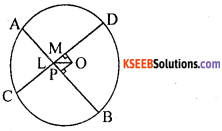OL ⊥ AB and OM ⊥ CD are drawn and OP is joined.
In ∆ OLP and ∆ OMP, since equal chords are at equal distance from centres.
OL = OM (OP common)
∠OLP = ∠OMP =90°
∆ OPL ≅ ∆ OPM. (R H S)
=> PL = PM [C.P.C.T]
But, AL = CM
=> AL – PL = CM – PM
=> AP = CP
Also, AB – AP = CD – CP
∴BP = DP
Thus, corresponding segments are equals.

Question 4.
If two interesting chords of a circle make equal angel with that: diameter passing through their point of intersecting. Prove that the chords are equal.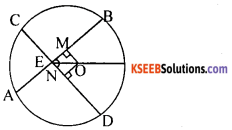OM ⊥ AB and ON⊥CD
In ∆ OME and ∆ ONEOE = OE (common)
∴ By A AS congruence
∆ OME ≅ ∆ ONE
OM = ON (C.P.C.T)
∴ AB = GD [Distance between the center to the chords equal.]
∴ Length of the chords are equal.

Question 5.
Two concentric circles of radii 13 cm and 5 cm are drawn. Find the length of the chord of the outer circle which touches the inner circle.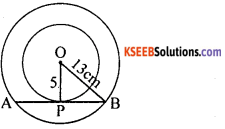In A OPB [P = 90°
OB2 = OP2 + BP2
(13)2 = (5)2 + BP2
BP2 = 165 – 25 = 144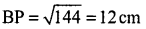AB = 2BP = 2 x 12 = 24cm

Question 6.
Tangents AP and AQ are drawn to circle with centre O, from an external point A. Prove that ∠PAQ = 2∠OPQ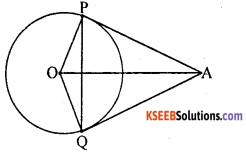‘O’ is the center of a circle.
AP & AQ are tangents.
In A POQ, OP = OQ [radius]Question 7.
Circles C1 and C2 touch internally at a point A and AB is a chord at the circle C1 intersecting C2 at P. Prove that AP = PB.Join OA, OP and OB.HYP OA = HYP OB [radii]
OP = OP [common]
∆ OAP ≅ ∆ OPB [by RHS theorem]
AP = PB [C.P.C.T]

Question 8.
A circle is touching the side BC of ∆ ABC at P. AB and AC when produced are touching the circle at Q and R respectively. Prove that AQ = V2 (Perimeter of ∆ ABC)AQ = AR → (1) [tangents drawn from A]
BP = BQ → (2) [tangents drawn from B]
CQ = CR → (3) [tangents drawn from C]
Perimeter of ∆ ABC = AB + BC + AC
= AB + BP + PC + AC
= AB + BQ + CR + AC
[From (1), (2) & (3)]
= AQ + AR
= AQ + AQ [∴ using(1)]
Perimeter of ∆ ABC = 2 AQ
AQ = 1/2 (Perimeter of ∆ ABC)

Question 9.
A straight line drawn through the point of contact of two circles with centres A and B intersect the circles at P and Q respectively. Show that AP and BQ are parallel.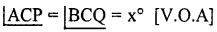In ∆ APC
AP = AC [radii of same circle]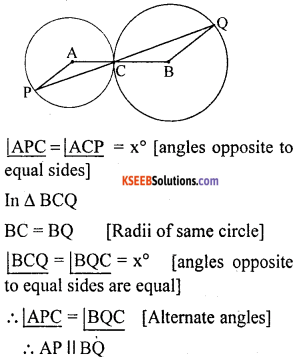Question 10.
Two circles with centres X and Y touch each other externally at P. Two diameters AB and CD are drawn one in each circle parallel to other. Prove that B, P and C are coliinear.